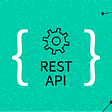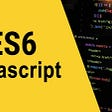# Background: Processing and p5.js

`float xLocation = 0;float yLocation = 0;void setup() {  background(255,255,255);  size(700,700);}void draw(){   float newXLocation = xLocation + random(100)-50;   float newYLocation = yLocation + random(100)-50;   if (newXLocation > 700) {     newXLocation = 700;   }   if (newXLocation < 0) {     newXLocation = 0;   }   if (newYLocation > 700) {     newYLocation = 700;   }   if (newYLocation < 0) {     newYLocation = 0;   }   //line(s.xLocation, s.yLocation, newXLocation, newYLocation);   stroke(255,255,255,0);   fill(random(255), random(255), random(255), 100);   float randomCircleSize = random(100);   ellipse(xLocation, yLocation, randomCircleSize, randomCircleSize);   xLocation = newXLocation;   yLocation = newYLocation;}`

# Math and Data Generation

## Block String Data

`lInvaders = ["".join(seq) for seq in itertools.product("10", repeat=15)] # all binary combinations of 0s and 1s and length 15`

## Rank Data

`lRanks = []for i in range(0,len(lInvaders)):        lRanks.append(lInvaders[i].count('1'))`
`counter=collections.Counter(lRanks)`
`| Rank |  Count | |------|--------| | 1    |  15    | | 2    |  105   | | 3    |  455   | | 4    |  1365  | | 5    |  3003  | | 6    |  5005  | | 7    |  6435  | | 8    |  6435  | | 9    |  5005  | | 10   |  3003  | | 11   |  1365  | | 12   |  455   | | 13   |  105   | | 14   |  15    | | 15   |  1     |`

## DataFrame and JSON Data Export

`oDataFrame = pd.DataFrame({'block_string': lInvaders, 'rank': lRanks})oDataFrame = oDataFrame.sort_values(by=['rank'], ascending=True)`
`oDataFrame.to_json(os.getcwd() + '/data/all_data.json', orient='records') # full datafor i, x in oDataFrame.groupby('rank'): # data per rank    x.to_json(os.getcwd() + '/data/data_rank_{}.json'.format(i), orient='records')`

## Complete Data Generation Code

`import itertoolsimport collections  import pandas as pdimport os  lInvaders = ["".join(seq) for seq in itertools.product('10', repeat=15)] # all binary combinations of 0s and 1s and length 15# now we can see how many invaders have what rank (number of times '1' occurs in a given invader string)lRanks = []for i in range(0,len(lInvaders)):        lRanks.append(lInvaders[i].count('1'))# put blocks string and rank into dataframeoDataFrame = pd.DataFrame({'block_string': lInvaders, 'rank': lRanks})oDataFrame = oDataFrame.sort_values(by=['rank'], ascending=True)oDataFrame.to_json(os.getcwd() + '/data/all_data.json', orient='records')# json for each rankfor i, x in oDataFrame.groupby('rank'):    x.to_json(os.getcwd() + '/data/data_rank_{}.json'.format(i), orient='records')# print for counts of each rank# counter=collections.Counter(lRanks) # print(counter)`

# Frontend Development

`for (int j=0;j<sz;j+=step) {  m = 1;  for (int i=0;i<sz/2;i+=step) {    c = (random(1) > .5)? 255:0; //black or white?    col[j][i]= c;    col[j][i+(sz-step)/m] = c;    m++;  }}`
`for (var j = 0; j < sz; j+=step) {  var m = 1;  for (var k = 0; k < sz / 2; k+=step) {    this.c = (s.random(1) > .5)? 255:0; //black or white?    this.col[j][k] = this.c;    this.col[j][k+(sz-step)/m] = this.c;    m++;  }}`
`for (var j = 0; j < sz; j+=step) {  var m = 1;  for (var k = 0; k < sz / 2; k+=step) {    this.c = (s.props.data[this.number].block_string.charAt(position) === "1") ? 255 : 0;  // either filled with rank color or white    position = position + 1;    this.col[j][k] = this.c;    this.col[j][k+(sz-step)/m] = this.c;    m++;  }}`
`...let number = 0;...for (var y = padding; y < s.height-2*padding; y += (sz+padding)) {  for (var x = padding; x < s.width-2*padding; x += (sz+padding)) {      if (number < iMaxInvaders) {      var invader = new Invader(x, y, number);      number = number + 1      invaders.push(invader);    }  }}`

## Color By Rank

`for (var j = 0; j < sz; j+=step) {  var m = 1;  for (var k = 0; k < sz / 2; k+=step) {    this.c = (s.props.data[this.number].block_string.charAt(position) === "1") ? aColors[s.props.data[this.number].rank - 1] : oBackgroundColor;  // either filled with rank color or white    position = position + 1;    this.col[j][k] = this.c;    this.col[j][k+(sz-step)/m] = this.c;    m++;  }}`
`const aColors = [s.color(132, 94, 194), s.color(214, 93, 177), s.color(255, 111, 145), s.color(255, 150, 113), s.color(255, 199, 95), s.color(249, 248, 113), s.color(44, 115, 210), s.color(0, 142, 155), s.color(0, 143, 122), s.color(155, 222, 126), s.color(75, 188, 142), s.color(3, 149, 144), s.color(28, 110, 125), s.color(47, 82, 107), s.color(26, 36, 120)]`

## The Last p5.js <-> React Caveat

`render() {  let aElements = [];  let canvasWidth = window.innerWidth > 690 ? 690 : window.innerWidth; // start width calculation for canvases at 690px, otherwise screen width  for (var i = 0; i < 15; i++) {    let canvasId = "canvas" + i.toString() + "-container";    aElements.push(      <div key={i}>        <h3>Invaders of Rank {i+1} ({aData[i].length.toString().replace(/\B(?=(\d{3})+(?!\d))/g, ",")} Total):</h3>        <P5Wrapper            p5Props={{ data: aData[i], canvasId, canvasWidth }}            onSetAppState={this.onSetAppState}        />      </div>    );  }  return (    ...  )  ...}`
`componentDidMount() {    this.canvas1 = new window.p5(sketch1, this.props.p5Props.canvasId)    this.canvas1.props = this.props.p5Props    this.canvas1.onSetAppState = this.props.onSetAppState}`
`<div    id={this.props.p5Props.canvasId}    style={{ width: "100%", textAlign: "center" }}/>`

# Footnotes

--

--

--

## More from Chris Frewin

https://reduxplate.com https://wheelscreener.com https://chrisfrew.in 👨‍💻 Full Stack Software Engineer 🏠 Austria/USA 🍺 Homebrewer ⛷🏃‍ 🚴 Outdoorsman

Love podcasts or audiobooks? Learn on the go with our new app.

## Library with Angular 6+: The ultimate guide## Everything You Need to Know About REST API## Jotai — Simple State Management for React## JavaScript ES6 Amazing Topics## Implementing Speech Recognition and Speech Synthesis## Real-Time Communication Apps## Dotenv or how to set up environment variables in your Nodejs project## Chris Frewin

https://reduxplate.com https://wheelscreener.com https://chrisfrew.in 👨‍💻 Full Stack Software Engineer 🏠 Austria/USA 🍺 Homebrewer ⛷🏃‍ 🚴 Outdoorsman

## “Nikyaa”React Clone — Construct week, Unit 4## I can’t install react using npx create-react-app. Here’s the solution — Let Me Fail## Journey of Cloning practo.com